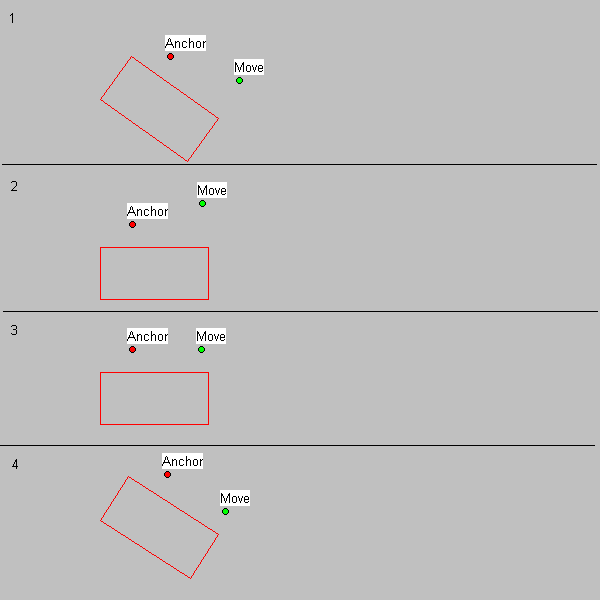﻿ L_AnnAdjustPoint | Function References | Raster Imaging C API Help
In This Topic ▼

# L_AnnAdjustPoint

#include "l_bitmap.h"

L_LTANN_API L_INT L_AnnAdjustPoint(pptAnchor, pptMove, dAngle, nType)

Modifies the point pptMove based on the angle from line (`pptAnchor`, `pptMove`) and the horizontal.

## Parameters

### pANNPOINT pptAnchor

Pointer to an ANNPOINT structure representing the anchor point.

### pANNPOINT pptMove

Pointer to an ANNPOINT structure representing the point to be adjusted

### L_DOUBLE dAngle

Angle of rotation in radians. Ranges from -Pi to Pi, (-3.1415 - 3.1415) where a positive angle corresponds to clockwise rotation.

### L_INT nType

Type of adjustment to point. Possible values are:

Type Value
ANNADJUST_HORIZONTAL 
ANNADJUST_VERTICAL 
ANNADJUST_45_DEGREES_FAVOR_X 
ANNADJUST_45_DEGREES_FAVOR_Y 

#### Returns

Value Meaning
SUCCESS The function was successful.
< 1 An error occurred. Refer to Return Codes.

## Comments

This utility function is used when creating custom annotation objects. It can be used to calculate the horizontal, vertical, or 45 degree component of a rotated annotation object. It can also be used to easily restrict the mouse cursor on rotated annotation objects.

As an example, consider a rectangle that is rotated 35 degrees in the clockwise direction.The user has clicked on the Anchor point, and moved the mouse to the Move point.

To find the horizontal component of the line that goes from (Anchor, Move), make the following call, and the ptMove point is adjusted to contain only the horizontal component.

The adjusted ptMove can be seen in step 4.

``L_AnnAdjustPoint(&ptAnchor, &ptMove, 35 * PI / 180, ANNADJUST_HORIZONTAL) ``

Conceptually, the following occurs:

1. Initial situation.

2. Points and rectangle are rotated 35 degrees counter clockwise.

3. Move point is adjusted to contain only the horizontal component.

4. Points and rectangle are rotated 35 degrees clockwise to give adjusted Move point.

Required DLLs and Libraries

Platforms

Win32, x64.

See Also

## Example

This is part of the custom annotations demo (from example1.c).
This handler gets called when there is a mouse move message on a user handle.
There are four user handles that are processed differently. The
HANDLE_ID_VERTICAL_LEFT and
HANDLE_ID_VERTICAL_RIGHT handles
restrict the mouse cursor to a line parallel to the top of the rectangle,
even if the rectangle is rotated. The
HANDLE_ID_HORIZONTAL_BOTTOM handle
restricts the mouse cursor to a line parallel to the side of the rectangle,
even if the rectangle is rotated. The
HANDLE_ID_HORIZONTAL_TOP handle
rotates the rectangle around the bottom handle (HANDLE_ID_HORIZONTAL_BOTTOM).

````/*<struct>*/ `
`#if !defined (LET_DEFINE)  `
`typedef struct _ANNCHILDDATA `
`{ `
`   HANNOBJECT  hObjectChange; `
`   POINT       pt0; `
`   POINT       pt; `
`   L_INT       nUserHandleID; `
`   HANNOBJECT  AnnObjectNeighbors; `
`}ANNCHILDDATA, *LPANNCHILDDATA; `
`#endif `
`/*</struct>*/ `
` `
`L_INT AnnAdjustPointExample(LPANNCHILDDATA pData, pANNMOUSEPOS pMousePos) `
`{ `
`   L_INT nRet; `
`   L_DOUBLE dAngle; `
`   ANNPOINT ptAnchor, ptMove; `
` `
`   nRet = L_AnnGetRotateAngle(pData->hObjectChange, &dAngle); `
`   if(nRet != SUCCESS) `
`      return nRet; `
` `
`   ptAnchor.x = pData->pt0.x; `
`   ptAnchor.y = pData->pt0.y; `
` `
`   ptMove.x = pMousePos->pt.x; `
`   ptMove.y = pMousePos->pt.y; `
` `
`   switch (pData->nUserHandleID) `
`   { `
`   case HANDLE_ID_VERTICAL_LEFT: `
`   case HANDLE_ID_VERTICAL_RIGHT: `
`      { `
`         L_DOUBLE dx, dy; `
`         POINT pt; `
` `
`         nRet = L_AnnAdjustPoint(&ptAnchor, &ptMove, dAngle, ANNADJUST_HORIZONTAL); `
`         if(nRet != SUCCESS) `
`            return nRet; `
`         dx = (ptMove.x - ptAnchor.x)/2; `
`         dy = (ptMove.y - ptAnchor.y)/2; `
` `
`         pt = pMousePos->pt; `
`         pt.x = (LONG)(ptAnchor.x + dx); `
`         pt.y = (LONG)(ptAnchor.y + dy); `
` `
`         nRet = L_AnnDefine(pData->hObjectChange, &pMousePos->pt, ANNDEFINE_APPEND);  `
`         if(nRet != SUCCESS) `
`            return nRet; `
`         nRet = L_AnnDefine(pData->AnnObjectNeighbors, &pt, ANNDEFINE_APPEND);  `
`         if(nRet != SUCCESS) `
`            return nRet; `
`      } `
`      break; `
` `
`   case HANDLE_ID_HORIZONTAL_BOTTOM: `
`      nRet = L_AnnAdjustPoint(&ptAnchor, &ptMove, dAngle, ANNADJUST_VERTICAL); `
`      if(nRet != SUCCESS) `
`         return nRet; `
`      nRet = L_AnnDefine(pData->hObjectChange, &pMousePos->pt, ANNDEFINE_APPEND);  `
`      if(nRet != SUCCESS) `
`         return nRet; `
`      nRet = L_AnnDefine(pData->AnnObjectNeighbors, &pMousePos->pt, ANNDEFINE_APPEND);  `
`      if(nRet != SUCCESS) `
`         return nRet; `
`      break; `
` `
`   case HANDLE_ID_HORIZONTAL_TOP: `
`      nRet = L_AnnDefine(pData->hObjectChange, &pMousePos->pt, ANNDEFINE_APPEND);  `
`      if(nRet != SUCCESS) `
`         return nRet; `
`      nRet = L_AnnDefine(pData->AnnObjectNeighbors, &pMousePos->pt, ANNDEFINE_APPEND);  `
`      if(nRet != SUCCESS) `
`         return nRet; `
`      break; `
` `
`   default: `
`      break; `
`   } `
` `
`   pMousePos->fUpdatePos = FALSE; `
`   if ( `
`      (pData->nUserHandleID == HANDLE_ID_VERTICAL_LEFT)       ||  `
`      (pData->nUserHandleID == HANDLE_ID_VERTICAL_RIGHT)      || `
`      (pData->nUserHandleID == HANDLE_ID_HORIZONTAL_TOP)      || `
`      (pData->nUserHandleID == HANDLE_ID_HORIZONTAL_BOTTOM)    `
`      ) `
`   { `
`      if (ISDIFFERENT(&ptMove,&pMousePos->pt)) `
`      { `
`         pMousePos->pt.x = ROUND(ptMove.x); `
`         pMousePos->pt.y = ROUND(ptMove.y); `
` `
`         pMousePos->fUpdatePos = TRUE; `
`      } `
`   } `
`   return SUCCESS; `
`} ````

Help Version 21.0.2021.4.7
Products | Support | Contact Us | Intellectual Property Notices
© 1991-2021 LEAD Technologies, Inc. All Rights Reserved.